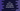# How to find the cube of a number using Macros in C++## How to find the cube of a number using Macros in C++:

Macros is a preprocessor directive in C or C++. This is a piece of code with a name. When the compiler finds that name in the code, it replaces that name with the name we have defined.

Macros helps us to write readable code and also using macros is easier than you think.

In this post, I will show you how we can use Macros in C++ to find the cube of a number. We will define one Macros that will work similar to a function and it will calculate the cube of a given number.

## C++ program:

Below is the complete program that uses a Macros to find the cube:

``````#include <iostream>
using namespace std;

#define CUBE(x) (x * x * x)

int main()
{
float num;

cout << "Enter a number: " << endl;
cin >> num;

cout << fixed << setprecision(2) << "Cube: " << CUBE(num) << endl;

return 0;
}``````

Here, CUBE is the Macros that takes one number and returns the cube of it. We are calling this Macros while calculating the cube of the number.

The number is stored in the float variable num. This is a user-input value and we are taking this value as input from the user. This value is passed to CUBE and its return value is printed on console.

We are using fixed and setprecision to make the output without any exponential part and upto two decimal places.

### Output:

If you run this program, it will print output as like below:

``````Enter a number:
100
Cube: 1000000.00

Enter a number:
123
Cube: 1860867.00``````